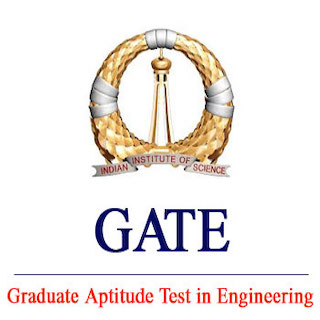## GATE 2019 General Aptitude (GA) Set-6

Q.1 – Q.5 carries one mark each.

Q.1 (MCQ)

A break down

B break

C breaks down

D breaks

Q.2 (MCQ)

A revolves

B plays

C sinks

D burst

Q.3 (MCQ)

A 1

B 2

C 3

D 6

Q.4 (MCQ)

A 666

B 3000

C 6000

D 12000

Q.5 (MCQ)

## A court is to a judge as _____________ is to a teacher.

A a student

B a punishment

C a syllabus

D a school

### The Answer is: D

Q.6 – Q.10 carries two marks each.

Q.6 (MCQ)

A P

B R

C S

D Q

Q.7 (MCQ)

## In the given diagram, teachers are represented in the triangle, researchers in the circle and administrators in the rectangle. Out of the total number of the people, the percentage of administrators shall be in the range of ___________.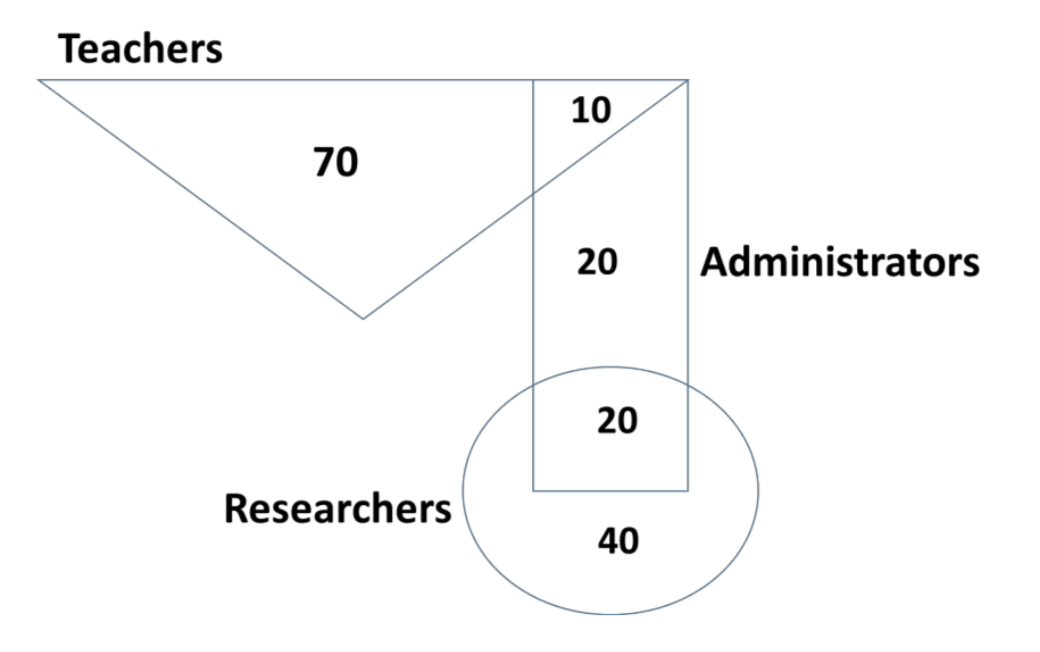A 0 to 15

B 16 to 30

C 31 to 45

D 46 to 60

Q.8 (MCQ)

## “A recent High Court judgement has sought to dispel the idea of begging as a disease — which leads to its stigmatization and criminalization — and to regard it as a symptom. The underlying disease is the failure of the state to protect citizens who fall through the social security net.”Which one of the following statements can be inferred from the given passage?

A Beggars are lazy people who beg because they are unwilling to work

B Beggars are created because of the lack of social welfare schemes

C Begging is an offence that has to be dealt with firmly

D Begging has to be banned because it adversely affects the welfare of the state

Q.9 (MCQ)

A 1000

B 975

C 900

D 225

Q.10 (MCQ)

## Three of the five students allocated to a hostel put in special requests to the warden. Given the floor plan of the vacant rooms, select the allocation plan that will accommodate all their requests.Request by X: Due to pollen allergy, I want to avoid a wing next to the garden. Request by Y: I want to live as far from the washrooms as possible, since I am very sensitive to smell.Request by Z: I believe in Vaastu and so want to stay in the South-west wing. The shaded rooms are already occupied. WR is washroom.

A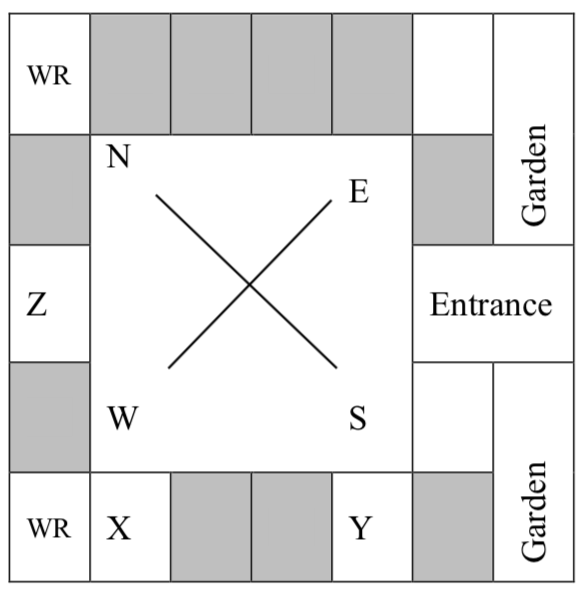B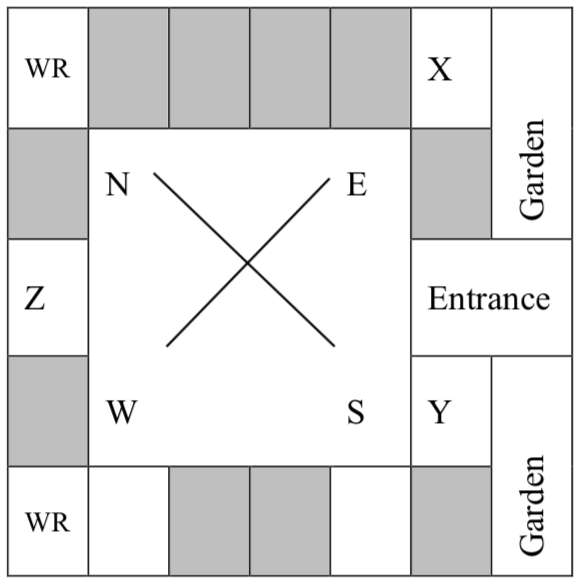C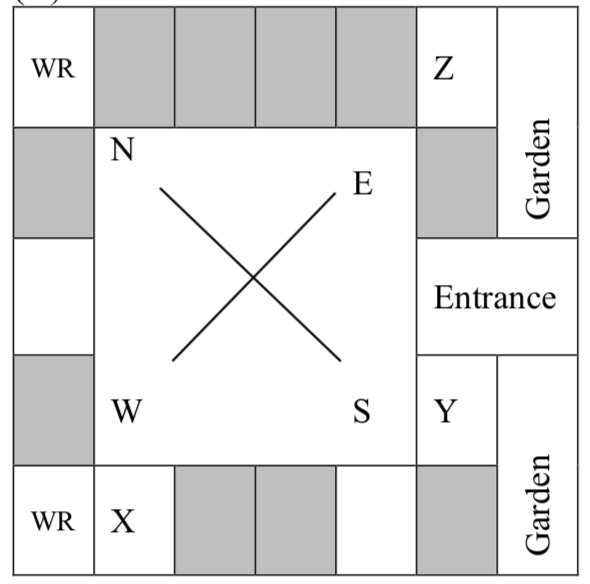D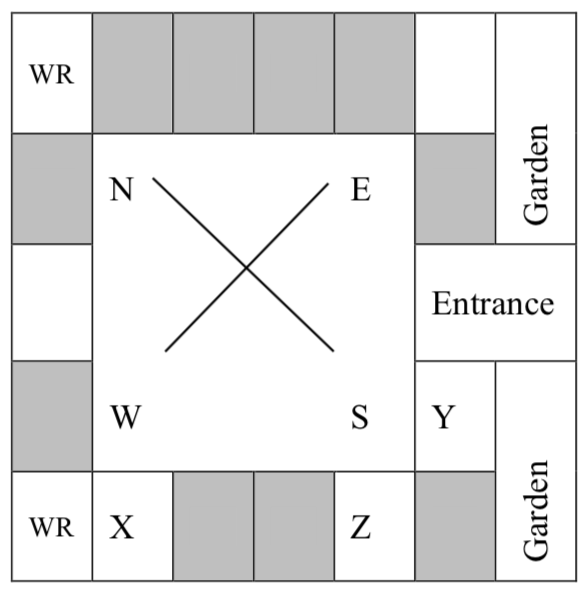## Computer Science and Information Technology Set-2

Q.1 – Q.25 carries one mark each.

Q.1 (MCQ)

## A certain processor uses a fully associative cache of size 16 kB. The cache block size is 16 bytes. Assume that the main memory is byte addressable and uses a 32-bit address. How many bits are required for the Tag and the Index fields respectively in the addresses generated by the processor?

A 24 bits and 0 bits

B 28 bits and 4 bits

C 24 bits and 4 bits

D 28 bits and 0 bits

Q.2 (MCQ)

## The chip select logic for a certain DRAM chip in a memory system design is shown below. Assume that the memory system has 16 address lines denoted by A15 to A0. What is the range of addresses (in hexadecimal) of the memory system that can get enabled by the chip select (CS) signal?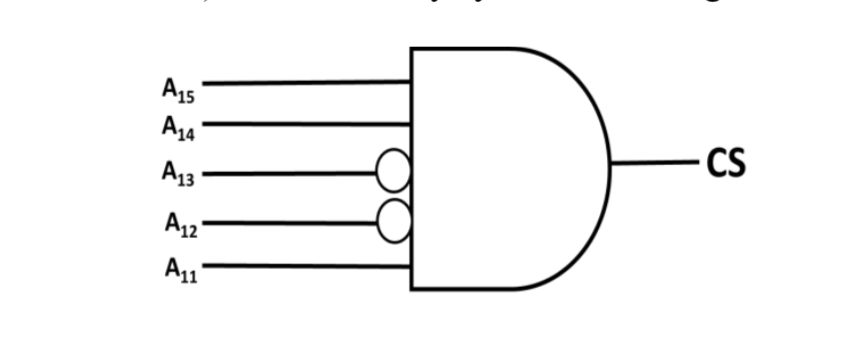A C800 to CFFF

B CA00 to CAFF

C C800 to C8FF

D DA00 to DFFF

Q.3 (MCQ)

## Which one of the following kinds of derivation is used by LR parsers?

A Leftmost

B Leftmost in reverse

C Rightmost

D Rightmost in reverse

Q.4 (MCQ)

## In 16-bit 2’s complement representation, the decimal number −28 is:

A 1111 1111 0001 1100

B 0000 0000 1110 0100

C 1111 1111 1110 0100

D 1000 0000 1110 0100

Q.5 (MCQ)

## Let 𝑈 = {1,2, ... , 𝑛}. Let 𝐴 = {(𝑥, 𝑋)|𝑥 ∈ 𝑋, 𝑋 ⊆ 𝑈}. Consider the following two statements on |𝐴|. I. |𝐴| = 𝑛2𝑛−1 II. |𝐴| = ∑𝑛𝑘=1k(𝑛𝑘)Which of the above statements is/are TRUE?

A Only I

B Only II

C Both I and II

D Neither I nor II

Q.6 (MCQ)

## Which one of the following is NOT a valid identity?

A (x⊕y)⊕z = x⊕(y⊕z)

B (x+y)⊕z = x⊕(y+z)

C x ⊕ y = x + y, if xy = 0

D x ⊕ y = (xy + x′y′)′

Q.7 (MCQ)

## If 𝐿 is a regular language over Σ = {𝑎, 𝑏}, which one of the following languages is NOT regular?

A 𝐿⋅𝐿R ={𝑥𝑦 |𝑥 ∈ 𝐿,𝑦R ∈𝐿}

B {𝑤𝑤R | 𝑤 ∈ 𝐿}

C Prefix(𝐿)={𝑥 ∈ 𝛴*|∃𝑦∈ 𝛴* such that 𝑥𝑦 ∈ 𝐿}

D Suffix(𝐿)={𝑦 ∈ 𝛴*|∃𝑥∈ 𝛴*such that 𝑥𝑦 ∈ 𝐿}

Q.8 (MCQ)

A 𝑛 bits

B 𝑛−1 bits

C 𝑛+1 bits

D 𝑛+2 bits

Q.9 (MCQ)

## Let 𝑋 be a square matrix. Consider the following two statements on 𝑋.I. 𝑋 is invertible. II. Determinant of 𝑋 is non-zero. Which one of the following is TRUE?

A I implies II; II does not imply I.

B II implies I; I does not imply II.

C I does not imply II; II does not imply I.

D I and II are equivalent statements.

Q.10 (MCQ)

## Let 𝐺 be an arbitrary group. Consider the following relations on 𝐺:𝑅1:∀𝑎,𝑏∈𝐺, 𝑎𝑅1𝑏 if and only if ∃𝑔∈𝐺 such that 𝑎=𝑔−1𝑏𝑔 𝑅2:∀𝑎,𝑏∈𝐺, 𝑎𝑅2𝑏 if and only if 𝑎=𝑏-1Which of the above is/are equivalence relation/relations?

A 𝑅1 and 𝑅2

B 𝑅1 only

C 𝑅2 only

D Neither 𝑅1 nor 𝑅2

Q.11 (MCQ)

## Consider the following two statements about database transaction schedules:I. Strict two-phase locking protocol generates conflict serializable schedules that are also recoverable.II. Timestamp-ordering concurrency control protocol with Thomas’ Write Rule can generate view serializable schedules that are not conflict serializable. Which of the above statements is/are TRUE?

A I only

B II only

C Both I and II

D Neither I nor II

Q.12 (MCQ)

A n!

B (𝑛 − 1)!

C 1

D (𝑛 − 1)!/2

Q.13 (MCQ)

## Compute \$\lim_{x\to 3}\frac{x^4-81}{2x^2-5x-3}\$

A 1

B 53/12

C 108/7

D Limit does not exist

Q.14 (MCQ)

## Which one of the following statements is NOT correct about the B+ tree data structure used for creating an index of a relational database table?

A B+ Tree is a height-balanced tree

B Non-leaf nodes have pointers to data records

C Key values in each node are kept in sorted order

D Each leaf node has a pointer to the next leaf node

Q.15 (MCQ)

A 3

B 5

C 9

D 24

Q.16 (MCQ)

A IMAP, POP3

B SMTP, POP3

C SMTP, MIME

D IMAP, SMTP

Q.17 (NAT)

## The following C program is executed on a Unix/Linux system:


#include <unistd.h>
int main()
{
int i;
for (i=0; i<10; i++)
if (i%2 == 0) fork(); return 0;
}


The total number of child processes created is ________.

31 to 31

Q.18 (NAT)

## Consider the following C program:


#include <stdio.h\>
int jumble(int x, int y){
x=2*x+y;
return x;
}
int main(){
int x=2, y=5;
y=jumble(y,x);
x=jumble(y,x);
printf("%d \n", x);
return 0;
}


The value printed by the program is ________.

26 to 26

Q.19 (NAT)